# SURFACE AREA Prisms and Cylinders Section 6 2

• Slides: 17SURFACE AREA Prisms and Cylinders Section 6 -2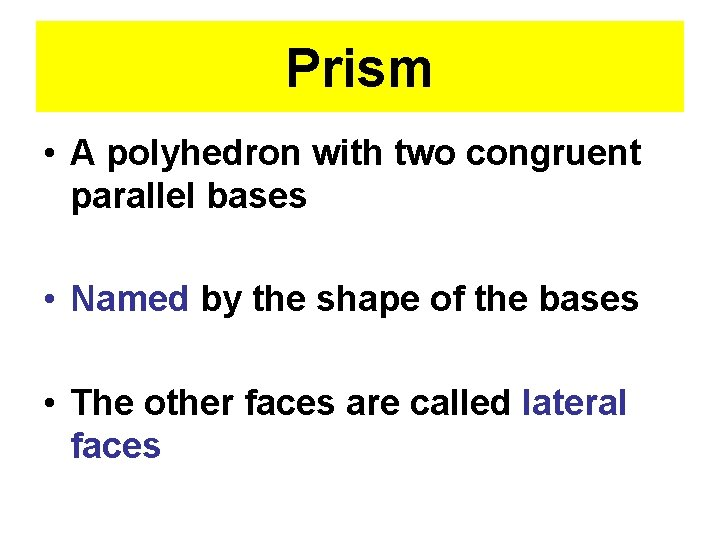Prism • A polyhedron with two congruent parallel bases • Named by the shape of the bases • The other faces are called lateral faces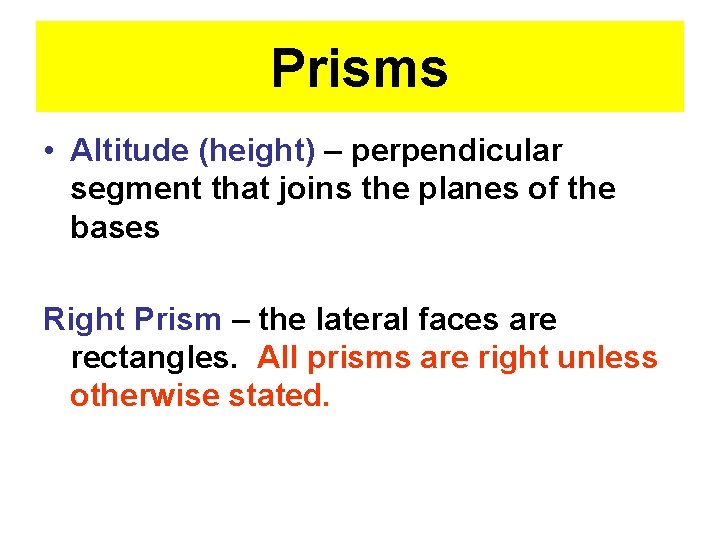Prisms • Altitude (height) – perpendicular segment that joins the planes of the bases Right Prism – the lateral faces are rectangles. All prisms are right unless otherwise stated.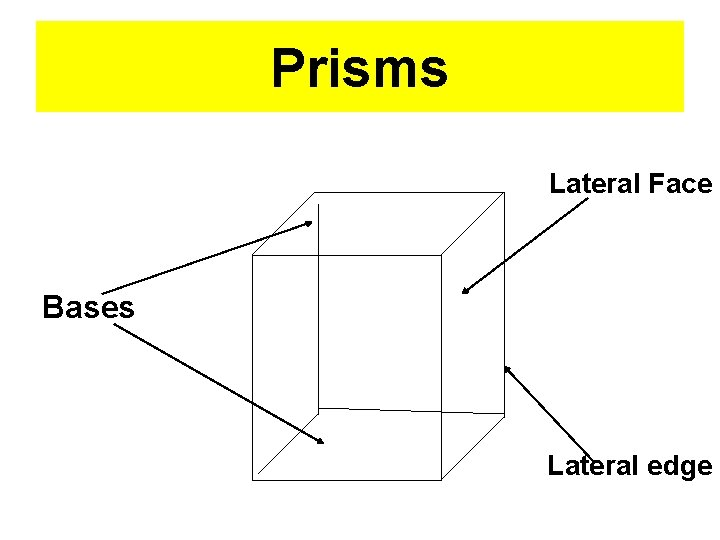Prisms Lateral Face Bases Lateral edge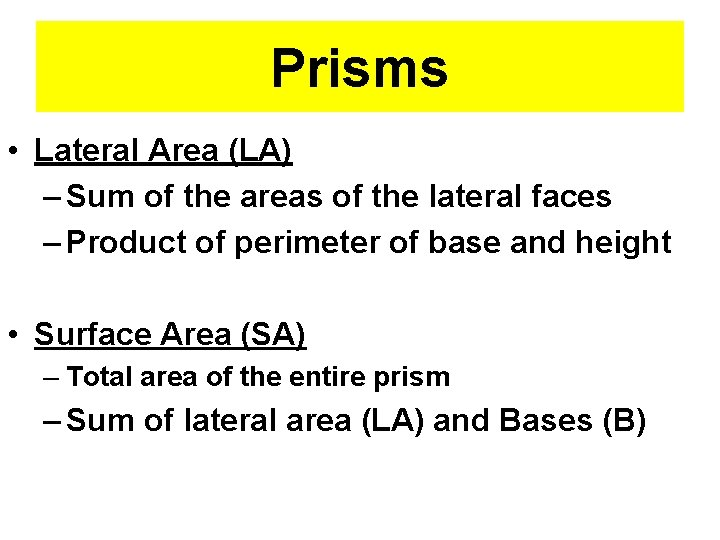Prisms • Lateral Area (LA) – Sum of the areas of the lateral faces – Product of perimeter of base and height • Surface Area (SA) – Total area of the entire prism – Sum of lateral area (LA) and Bases (B)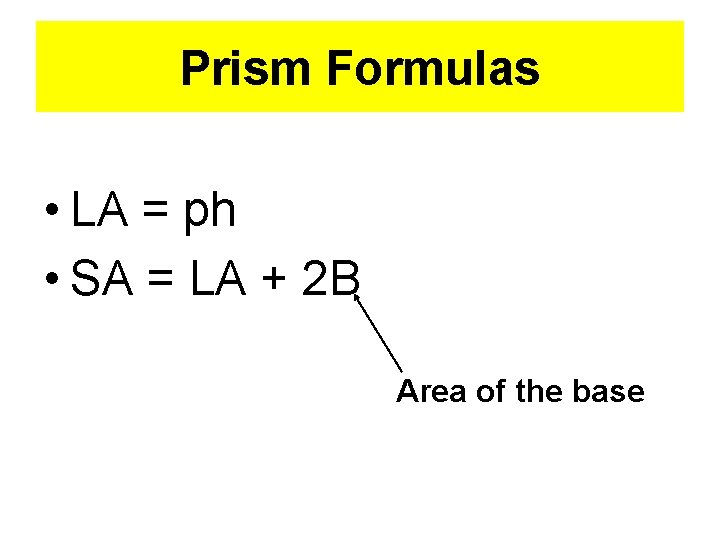Prism Formulas • LA = ph • SA = LA + 2 B Area of the baseCYLINDERS • Is like a prism but the bases are circles • Altitude (height) – Perpendicular segment that joins the planes of the bases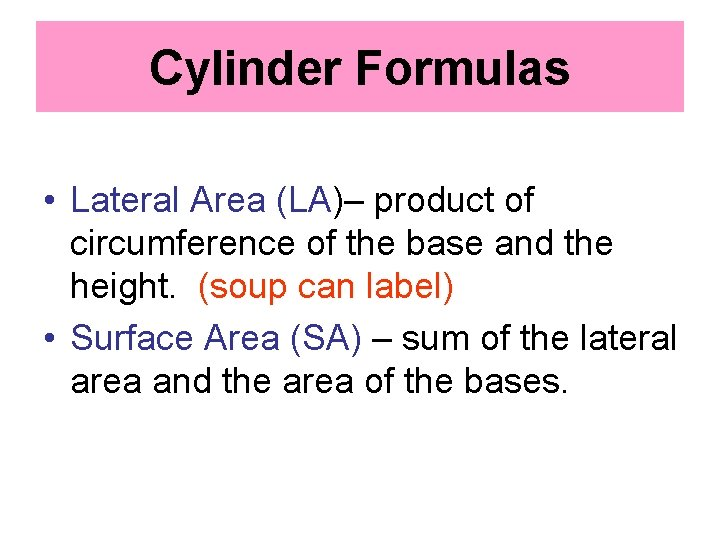Cylinder Formulas • Lateral Area (LA)– product of circumference of the base and the height. (soup can label) • Surface Area (SA) – sum of the lateral area and the area of the bases.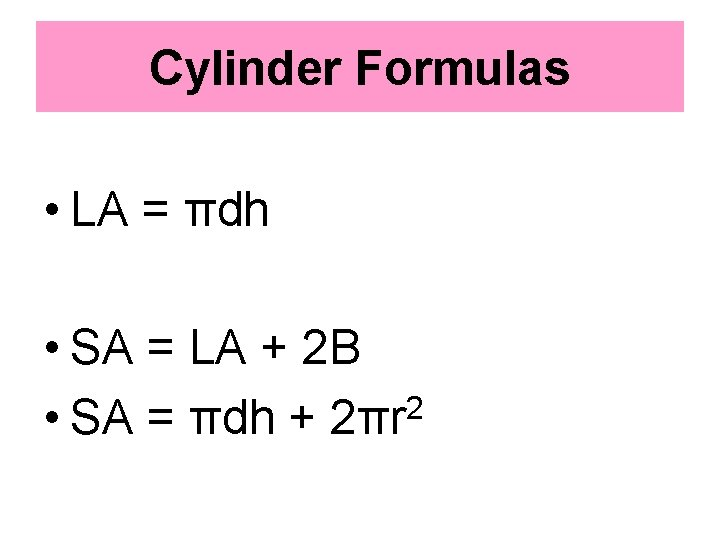Cylinder Formulas • LA = πdh • SA = LA + 2 B 2 • SA = πdh + 2πrFind LA and SA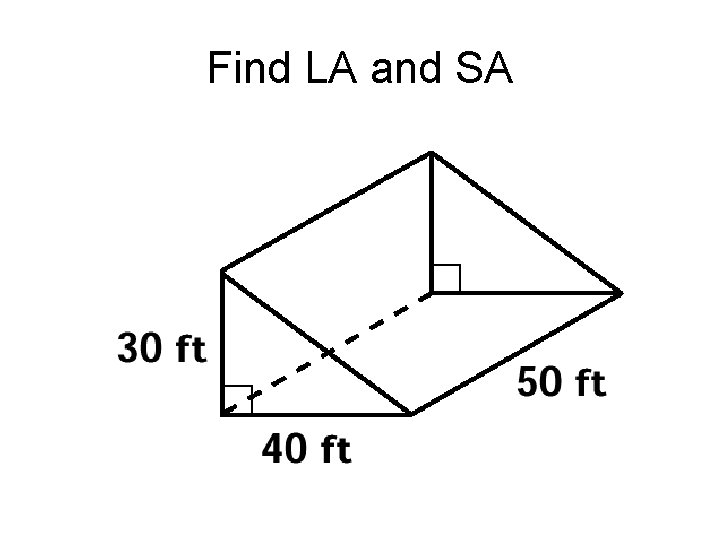Find LA and SA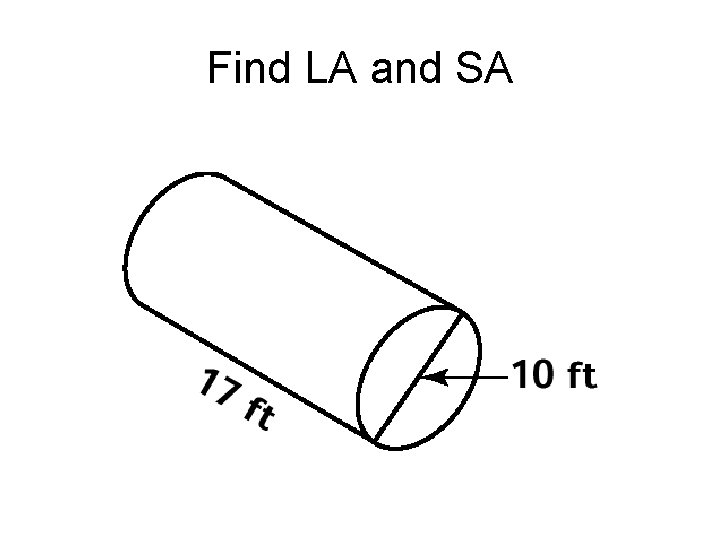Find LA and SAFind LA and SA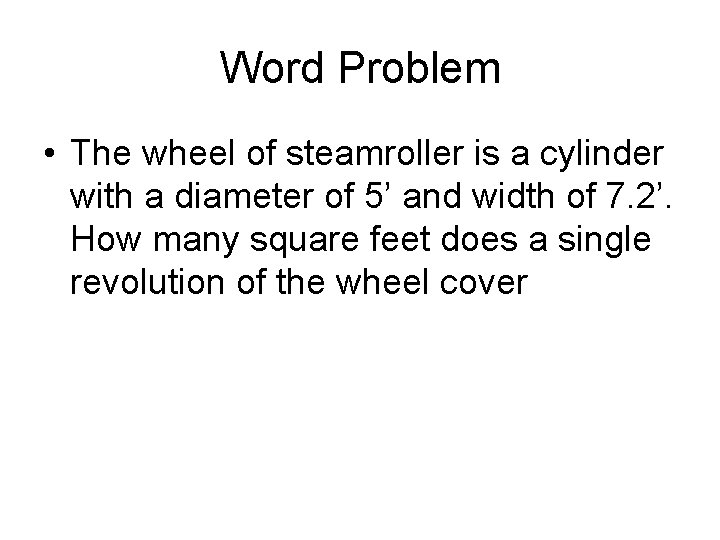Word Problem • The wheel of steamroller is a cylinder with a diameter of 5’ and width of 7. 2’. How many square feet does a single revolution of the wheel cover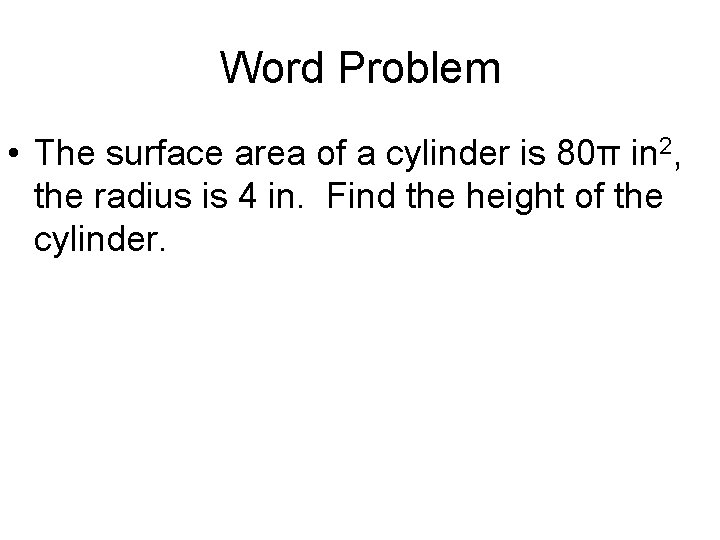Word Problem • The surface area of a cylinder is 80π in 2, the radius is 4 in. Find the height of the cylinder.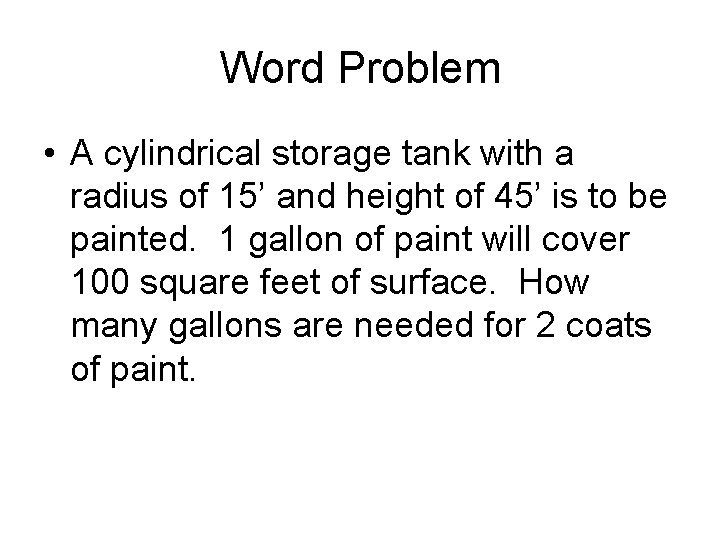Word Problem • A cylindrical storage tank with a radius of 15’ and height of 45’ is to be painted. 1 gallon of paint will cover 100 square feet of surface. How many gallons are needed for 2 coats of paint.Word Problem • A right triangular prism has a height of 5 cm, its base is an equilateral triangle with a side of 2 cm. Find the LA and SA